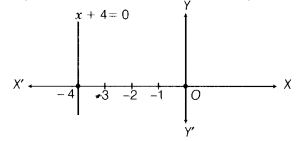# How many solution(s) of the equation 2x + 1 = x -3 are there on the (i)number line?

How many solutions) of the equation 2x + 1 = x -3 are there on the
(i) number line?
(ii) cartesian plane?

Given equation is 2x + 1 = x - 3
=> 2x - x = - 3 - 1
∴ x = -4…(i)
(i) Number line represents the all real values of x on the X-axis. Therefore, x = - 4 is exactly one point which lies on the number line.
(ii) If we treated x = -4 as an equation in two variables, then it can be written as 1.x+0.y=-4, where as the equation x + 4 = 0 represents a straight line parallel to Y-axis and infinitely many points lie on a line in the cartesian plane.